Courses

# Work, Power And Energy NAT Level - 2

## 10 Questions MCQ Test Basic Physics for IIT JAM | Work, Power And Energy NAT Level - 2

Description
This mock test of Work, Power And Energy NAT Level - 2 for Physics helps you for every Physics entrance exam. This contains 10 Multiple Choice Questions for Physics Work, Power And Energy NAT Level - 2 (mcq) to study with solutions a complete question bank. The solved questions answers in this Work, Power And Energy NAT Level - 2 quiz give you a good mix of easy questions and tough questions. Physics students definitely take this Work, Power And Energy NAT Level - 2 exercise for a better result in the exam. You can find other Work, Power And Energy NAT Level - 2 extra questions, long questions & short questions for Physics on EduRev as well by searching above.
*Answer can only contain numeric values
QUESTION: 1

### In the figure shown initially spring is in unstretched state and blocks are at rest. Now 100N force is applied on block A and B as shown in the figure. After some time velocity of A becomes 2m/s and that of B is 4m/s and block A displaced by amount 10cm and spring is stretched by amount 30cm. Then work done by spring (in Joule) force on A will be :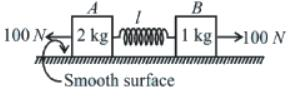Solution: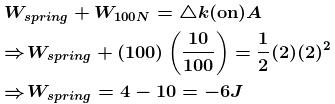*Answer can only contain numeric values
QUESTION: 2

### Starting at rest, a 5kg object is acted upon by only one force as indicated in the figure. Find the total work done (in Joule) by the force on the object :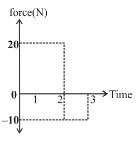Solution: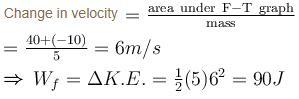*Answer can only contain numeric values
QUESTION: 3

### A particle is projected vertically upwards with a speed of 16m/s, after some time, when it again passes through the point of projection, its speed is found to be 8m/s. It is known that the work done by air resistance is same during upward and downward motion. Then the maximum height (in m) attained by the particle is : (Take g = 10m/s2) :

Solution:

From work energy theorem for upward motion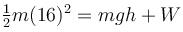(work by air resistance) for downward motion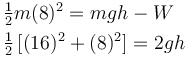or  h = 8m

*Answer can only contain numeric values
QUESTION: 4

A particle A of mass 10/7 kg is moving in the positive x direction. Its initial position is x = 0 and initial velocity is 1m/s. The velocity (in m/s) at x = 10 is ......... .

(use the graph given)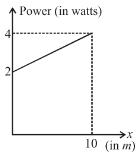Solution:

Area under P-x graph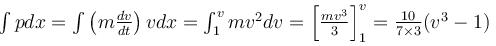From graph;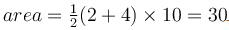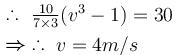*Answer can only contain numeric values
QUESTION: 5

The blocks of mass m1 = 1kg and m2 = 2kg are connected by a spring, rest on a rough horizontal surface. The spring is unstretched. The spring constant of spring is K = 2N/m. The coefficient of friction between blocks and horizontal surface is μ = 1/2. Now the left block is imparted a velocity u towards right as shown. Then what is the largest value of u (in m/s) such that the block of mass m2 never moves. (Take g = 10 m/s2)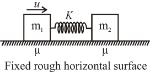Solution:

For the block of mass m2, not to move, the maximum compression in the spring x0 should be such that

kx0 = μm      .....(i)

Applying work energy theorem to block of mass m1 we get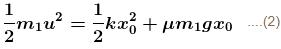From equation (1) and (2) we get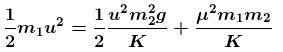putting the appropriate value we get μ  = 10m/s.

*Answer can only contain numeric values
QUESTION: 6

A block of mass m is attached with a massless spring of force constant k. The block is placed over a fixed rough inclined surface for which the coefficient of friction is μ = 3/4. The block of mass m is initially at rest. The block of mass M is released from rest with spring in unstretched state. The minimum value of M required to move the block up the plane is given by λm  Find the value of  λ.

(neglect mass of string and pulley and friction in pulley.)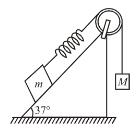Solution:

As long as the block of mass m remains stationary, the block of mass M released from rest comes down by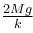(before coming it rest momentarily again).

Thus the maximum extension in spring is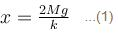for block of mass m to just move up the incline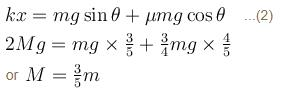*Answer can only contain numeric values
QUESTION: 7

In the given figure, the magnitude of work done by frictional force on upper and lower block?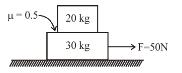Solution:

Assume 20kg and 30kg block move together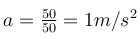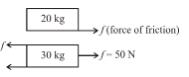∴  friction force on 20kg block is  f = 20 × 1 = 20N

The maximum value of frictional force is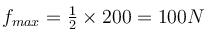Hence no slipping is occurring.

∴ The value of frictional force is f = 20N

Distance traveled in t = 2 seconds.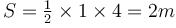Work done by frictional force on upper block is
Wfriction = 20 × 2 = 40J
Work done by friction force on lower block is  = –20 × 2 = –40J

*Answer can only contain numeric values
QUESTION: 8

A fire hose has a diameter of 2.5cm and is required to direct a jet of water to a height of at least 40m. The minimum power (in kW) of the pump needed for this hose is :

Solution:

The speed of the water leaving the hose must be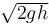if it is to reach a height h when directed  vertically upward. If the diameter is d, the volume of water ejected at this speed is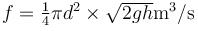⇒ Mass ejected is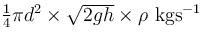This kinetic energy of this  water leaving the hose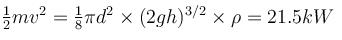*Answer can only contain numeric values
QUESTION: 9

If there is no friction any where, the speed of the wedge, as the block leaves the wedge is :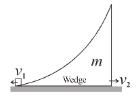Solution:

Linear momentum is conserved only in horizontal direction.
mv1=mv2…..(i)
1/2(mv1)2+1/2(mv2)= mgh....(ii)
From (i)&(ii),
v2= 10ms-1

*Answer can only contain numeric values
QUESTION: 10

A spring lies along an x-axis attached to a wall at one end and a block at the other end. The block rests on a frictionless surface at x = 0. A force of constant magnitude F is applied to the block that begins to compress the spring, until the block comes to a maximum displacement xmax.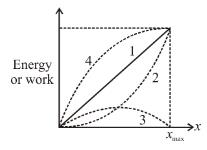During the displacement, which of the curves shown in the graph best represents the kinetic energy of the block.

Solution:

Applying W-E theorem on the block for any compression x :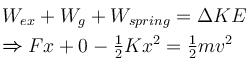⇒ KE vs x is inverted parabola.